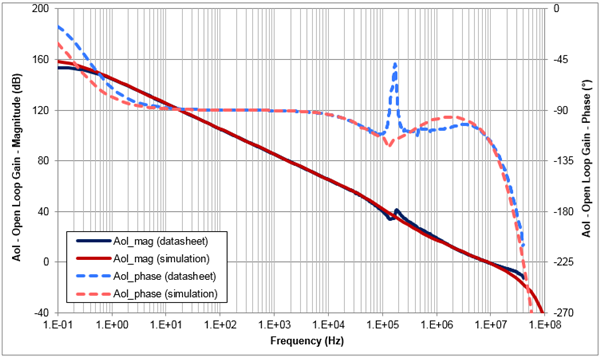# “Trust, but verify” SPICE model accuracy, part 5: input offset voltage and open-loop gain

Previous installments of this blog post series discussed the need to verify SPICE model accuracy and how to measure common-mode rejection ratio (CMRR), offset voltage versus common-mode voltage (Vos vs. Vcm), slew rate (SR) and open-loop output impedance (Zo). In part 5, I’ll explain how to verify two of the most impactful specs of precision operational amplifiers (op amps): input offset voltage (Vos) and open-loop gain (Aol).

Input offset voltage

Vos is the difference in voltage between an op amp’s two input pins. Typical offset voltages range from millivolts down to nanovolts, depending on the device. Vos adds in series with any externally applied input voltage (Vin), and therefore can cause errors if Vos is significant compared to Vin. For this reason, op amps with low Vos are highly desirable for precision circuits with small input voltages.

Figure 1 shows the application of a 1mV input voltage to an op amp with Vos equal to 0.1mV. Because Vos is 10% of Vin, the offset voltage contributes a 10% error in the overall circuit output. While this is a fairly extreme example, it shows the impact that Vos can have on op amp designs.Figure 1: Input offset voltage contribution to DC error

To measure the Vos of an op amp, configure the op amp as a unity gain buffer with its noninverting input connected to mid supply (ground in split-supply circuits). Wire a differential voltage probe between the op amp input pins, and make sure to match the power-supply voltage and common-mode voltage conditions given in the op amp data sheet. Figure 2 shows the recommended test circuit.Figure 2: Input offset voltage test circuit

Let’s use this circuit to measure the Vos of the OPA189, a new zero-drift, low-noise amplifier from TI. Simply run a DC analysis and observe the voltage at probe Vos, as shown in Figure 3.Figure 3: OPA189 Vos result

The measured input offset voltage is -400nV, or -0.4µV. This correlates exactly with the spec in the OPA189 data sheet.

Open-loop gain

An op amp’s open-loop gain is arguably its most important parameter, affecting nearly all aspects of linear or small-signal operation including gain bandwidth, stability, settling time and even input offset voltage. For this reason, it’s essential to confirm that your op amp SPICE model matches the behavior given in the device’s data sheet. Figure 4 shows the recommended test circuit.Figure 4: Open loop gain test circuit

This test circuit is very similar to the one used to measure open-loop output impedance. Inductor L1 creates closed-loop feedback at DC while allowing for open-loop AC analysis, and capacitor C1 shorts the inverting input to signal source Vin at AC in order to receive the appropriate AC stimulus.

As explained by Bruce Trump in his classic blog post, “Offset Voltage and Open-Loop Gain – they’re cousins,” you can think of Aol as an offset voltage that changes with DC output voltage. Therefore, to measure Aol, run an AC transfer function over the desired frequency range and plot the magnitude and phase of Vo/Vos. Make sure to match the specified data sheet conditions for power-supply voltage, input common-mode voltage, load resistance and load capacitance.

Let’s use this method to test the Aol of the OPA189.Figure 5: OPA189 Aol result

In this case, the op amp’s Aol is modeled very closely to the data sheet spec. The spike in the data sheet’s Aol around 200kHz is caused by the chopping network at the input of the amplifier and is not modeled, although its effect on the nearby magnitude and phase response is.

Thanks for reading this fifth installment of the “Trust, but verify” blog series! In the sixth and final installment, I’ll discuss how to measure op amp voltage and current noise. If you have any questions about simulation verification, log in and leave a comment, or visit the TI E2E™ Community Simulation Models forum.